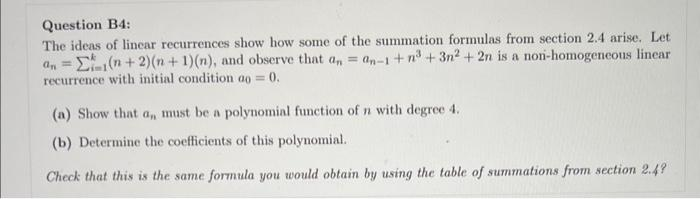# Question Question B4: The ideas of linear recurrences show how some of the summation formulas from section 2.4 arise. Let $$a_{n}=\sum_{i=1}^{k}(n+2)(n+1)(n)$$, and observe that $$a_{n}=a_{n-1}+n^{3}+3 n^{2}+2 n$$ is a non-homogeneous linear recurrence with initial condition $$a_{0}=0$$. (a) Show that $$a_{n}$$ must be a polynomial function of $$n$$ with degree 4 . (b) Determine the coefficients of this polynomial. Check that this is the same formula you would obtain by using the table of summations from section $$2.4 ?$$Transcribed Image Text: Question B4: The ideas of linear recurrences show how some of the summation formulas from section 2.4 arise. Let $$a_{n}=\sum_{i=1}^{k}(n+2)(n+1)(n)$$, and observe that $$a_{n}=a_{n-1}+n^{3}+3 n^{2}+2 n$$ is a non-homogeneous linear recurrence with initial condition $$a_{0}=0$$. (a) Show that $$a_{n}$$ must be a polynomial function of $$n$$ with degree 4 . (b) Determine the coefficients of this polynomial. Check that this is the same formula you would obtain by using the table of summations from section $$2.4 ?$$
Transcribed Image Text: Question B4: The ideas of linear recurrences show how some of the summation formulas from section 2.4 arise. Let $$a_{n}=\sum_{i=1}^{k}(n+2)(n+1)(n)$$, and observe that $$a_{n}=a_{n-1}+n^{3}+3 n^{2}+2 n$$ is a non-homogeneous linear recurrence with initial condition $$a_{0}=0$$. (a) Show that $$a_{n}$$ must be a polynomial function of $$n$$ with degree 4 . (b) Determine the coefficients of this polynomial. Check that this is the same formula you would obtain by using the table of summations from section $$2.4 ?$$&#12304;General guidance&#12305;The answer provided below has been developed in a clear step by step manner.Step1/3Given that: The non-homogeneous linear recurrence $$\mathrm{{a}_{{n}}={a}_{{{n}-{1}}}+{n}^{{3}}+{3}{n}^{{2}}+{2}{n}}$$with the initial condition $$\mathrm{{a}_{{0}}={0}}$$The objective is to determine,(a). Show that $$\mathrm{{a}_{{n}}}$$must be a polynomial function of $$\mathrm{{n}}$$ with degree $$\mathrm{{4}}$$.(b). Determine the coefficient of this polynomial.In this problem, the characteristic polynomial equation associated with the homogeneous recurrence relation. So, use the iteration or the formula to find the require values.Explanation:Please refer to solution in this step.Step2/3Solving for (a),The recurrence relation for $$\mathrm{{a}_{{n}}}$$ is non-homogeneous, with a particular solution given by the degree-4 polynomial $$\mathrm{{p}{\left({n}\right)}={a}{n}^{{4}}+{b}{n}^{{3}}+{c}{n}^{{2}}+{d}{n}+{e}}$$.Since the degree of the polynomial is $$\mathrm{{4}}$$, Now, need to find $$\mathrm{{5}}$$ constants to fully specify $$\mathrm{{p}{\left({n}\right)}}$$ and therefore $$\mathrm{{a}_{{n}}}$$. Now, obtain these constants by solving for the coefficients of $$\mathrm{{p}{\left({n}\right)}}$$ using the initial condition $$\mathrm{{a}_{{0}}={0}}$$ and the recurrence relation $$\mathrm{{a}_{{n}}={a}_{{{n}-{1}}}+{n}^{{3}}+{3}{n}^{{2}}+{2}{n}}$$.Hence, $$\mathrm{{a}_{{n}}}$$must be a polynomial function of $$\mathrm{{n}}$$ with degree $$\mathrm{{4}}$$.Explanation:In this step, the solution of non homogeneous part $$\mathrm{{p}{\left({n}\right)}}$$ known as the particular solution.Explanation:Please refer to solution in this step.Step3/3Solving for (b),Using the recurrence relation and initial condition,$$\mathrm{{a}_{{1}}={a}_{{0}}+{1}^{{3}}+{3}{\left({1}^{{2}}\right)}+{2}{\left({1}\right)}={6}}$$$$\mathrm{{a}_{{2}}={a}_{{1}}+{2}^{{3}}+{3}{\left({2}^{{2}}\right)}+{2}{\left({2}\right)}={26}}$$\( \mathrm{{a}_{{3}}={ ... See the full answer